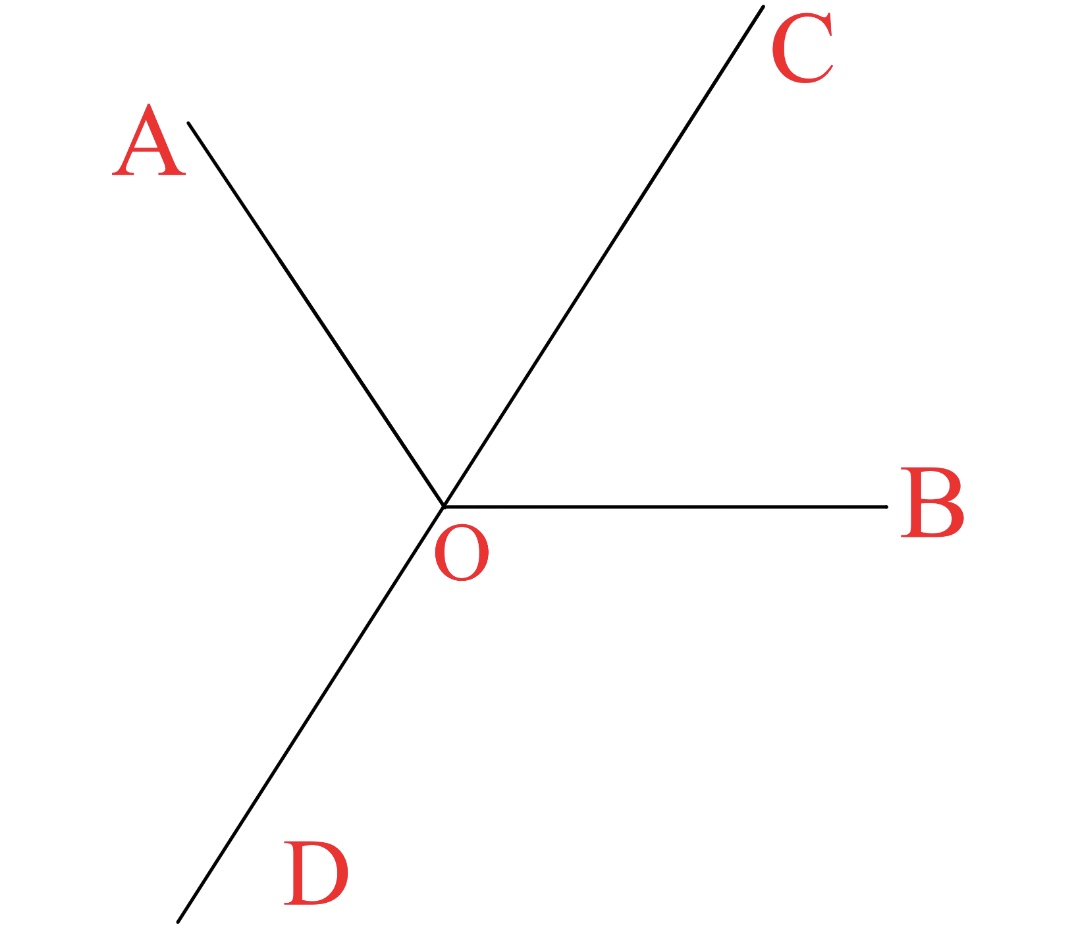# Problem Set: what 13-year old kids do in China

In this note, I will provide a set of problems, all copied from my homework or test assignments. I am now 13 but I suppose there are some interesting problems in my homework anyways. Why not take a look?

Assumptions(all assumptions are made from the knowledge level of Secondary 1, which is Grade 7, in China)(Just to brag, I know integration now :) ) :

• Imaginary and complex numbers do not exist.
• There is no angle greater than $180^\circ$ or less than $0^\circ$. No angle equals $180^\circ$ or $0^\circ$.
• This must be explained further:• Here $OC$ bisects $\angle AOB$ while $OD$ is not considered as a bisector at all.
• Angle bisectors are rays. Rays, straight lines and line segments are to be STRICTLY DISTINGUISHED FROM ONE ANOTHER.

Part 1
Part 2
Part 3
Part 4
Part 5
$\color{#D61F06}\small \text{Note created on 2021.3.11, three days to}~ \pi ~\text{day}$Note by Jeff Giff
4 months, 3 weeks ago

This discussion board is a place to discuss our Daily Challenges and the math and science related to those challenges. Explanations are more than just a solution — they should explain the steps and thinking strategies that you used to obtain the solution. Comments should further the discussion of math and science.

When posting on Brilliant:

• Use the emojis to react to an explanation, whether you're congratulating a job well done , or just really confused .
• Ask specific questions about the challenge or the steps in somebody's explanation. Well-posed questions can add a lot to the discussion, but posting "I don't understand!" doesn't help anyone.
• Try to contribute something new to the discussion, whether it is an extension, generalization or other idea related to the challenge.

MarkdownAppears as
*italics* or _italics_ italics
**bold** or __bold__ bold
- bulleted- list
• bulleted
• list
1. numbered2. list
1. numbered
2. list
Note: you must add a full line of space before and after lists for them to show up correctly
paragraph 1paragraph 2

paragraph 1

paragraph 2

[example link](https://brilliant.org)example link
> This is a quote
This is a quote
    # I indented these lines
# 4 spaces, and now they show
# up as a code block.

print "hello world"
# I indented these lines
# 4 spaces, and now they show
# up as a code block.

print "hello world"
MathAppears as
Remember to wrap math in $$ ... $$ or $ ... $ to ensure proper formatting.
2 \times 3 $2 \times 3$
2^{34} $2^{34}$
a_{i-1} $a_{i-1}$
\frac{2}{3} $\frac{2}{3}$
\sqrt{2} $\sqrt{2}$
\sum_{i=1}^3 $\sum_{i=1}^3$
\sin \theta $\sin \theta$
\boxed{123} $\boxed{123}$

Sort by:

I think by rods you mean rays, maybe they teach a different terminology in China

- 4 months, 3 weeks ago

yup

- 4 months, 3 weeks ago

i edited that just now :)

- 4 months, 3 weeks ago